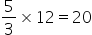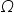Physics
Easy

Question

# Two wires of the same material have the same length, but their radii are in the ratio of 5:3. They are combined in series, where the resistance of the thicker wire is 12 ohms. Calculate the total resistance of the combination

## 40 Ω12 Ω33.3 Ω0.03 ΩHint:

## The correct answer is: 33.3 Ω

### When two wires of radii in the ratio of 5:3 are combined in series, where the resistance of the thicker wire is 12 ohms, the resistance of the combination is 32 Ω. Given, the radii of the wire are in the ratio of 5:3 which is R2/R1 We can write it as, R2/R1= 5/3, where R2= (5/3) R1 The R1 given is the thicker wire with resistance of 12 Ω. So R2=Hence, their total resistance is RT= R1+R2, i.e. 12+20= 32 Ω#### With Turito Foundation.#### Get an Expert Advice From Turito.